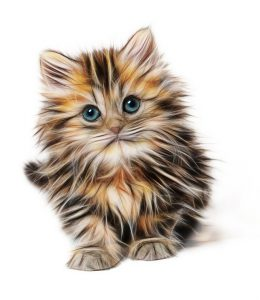Want to share your content on R-bloggers? click here if you have a blog, or here if you don't.Let’s make data
R is good a making simulated data sets. These data sets are useful for learning programming.
Instead of having to spend all your time cleaning up your data you have data ready to use for learning how to program.
The data that will be generated here will be about cats, toys and blocks. The data will be used in lessons about ggplot2 later on.

Answers to the exercises are available here.

Exercise 1
R has function for making distributions.
What command will list the distributions that R knows how to use?
Read the help file on Distributions.

Exercise 2
Now we are going to use the distribution function to generate data.
First we are going to make this data reproducible by setting seed
Finish this command by choosing a number for the seed.
`set.seed()`

Exercise 3

Next we will make an object called Lbody about the length of cat’s body.
This will be a continuous variable measured in inches. Change the parameters to another unit of measurement
`Lbody <- rnorm(100, mean = 18, sd =3)`

Also we are going to weigh the cats. Change this code to your unit of measurement.

`weightslbs <- rnorm(100, mean = 10,sd =3)`

Exercise 4
What does rnorm do?

Exercise 5
I measured a very large cat to get a mean of 18 inches. Change the code get lengths of cats in centimeters based on what you think the mean and standard deviation should be.
Also change the code to what you think the mean age of cats is.
`Age_of_cat <- rnorm(100, mean =12, sd =3)`

Exercise 6
Now go back and change the distribution of the cats age to any distribution you would like.

Exercise 7
#Another way to generate data is to use sample

`hasClaws <- sample(c(TRUE, FALSE),size=100, replace=T)`

“c” is combine or concatenate
Sample takes 100 samples of true or false

Write code to generate a sample of 100 cats with or without a tail
Sample also take parameters like int

Write code using function `sample.int` to generate 100 cats that have five different colors of coats

Exercise 8

The cats have blocks. Write code to generate blocks for the cats.
If you wish name your blocks as regular polyhedron

Write code to generate bells for the cats

The cats like toys.
Write code to generate toys for cats

Exercise 9

Write code for two more variables, an id variable and a grouping variable of small, medium and large.

Exercise 10

Place all the variables into a dataframe.